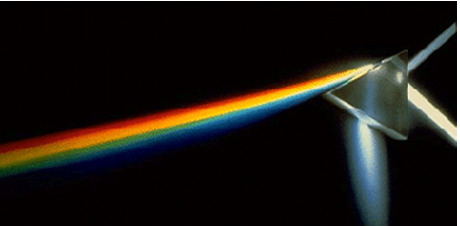# Critical angleThe critical angle is the angle of incidence above which total internal reflection occurs. If the speed of light is $1.5 \times 10^8\text{ m/s}$ in a particular medium, then what is the critical angle of the light passing through this medium into the air?

The speed of light in the air is $3.0 \times 10^8\text{ m/s}.$

×